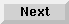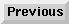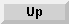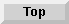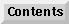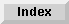10 Matrix Operations

## Array Functions

### System Functions

The function statement causes each element in the operand array to be evaluated by the specified function. The result becomes the corresponding element of the result array. Syntax for this statement is as follows:

MAT result array = function (operand array)

The function must be a single argument system function like ABS or SQR. User defined functions are not allowed.

For example, the following program line assigns the square root of each element in array A to the corresponding element in array B:

```100   MAT B=SQR(A)
```

### The SUM Function

The SUM function returns the sum of all elements in an array.

SUM operand array

The following two sequences are equivalent:

```10 M A(10,10)=SUM A              10   FOR J=1 TO 10
20 I=SUM A                       20     FOR K=1 TO 10
30       I=I+A(J,K)
40     NEXT K
50   NEXT J
```

### The ROW Function

The ROW function returns the number of rows in the array according to its current working size. The number of rows corresponds to the subscript which is second from the right.

ROW operand array

### The COL Function

The column function returns the number of columns in the array according to its current working size. The number of columns corresponds to the rightmost subscript.

COL operand array

Eloquence Language Manual - 19 DEC 2002